# Solution assignment 05 Optimizing f(x)

### Assignment 5

Find the extremes of the function: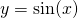on the interval interval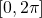.

### Solution

The derivative of the function is: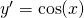The equation: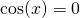has the following solutions in the given interval: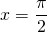or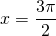When we look at the graph of: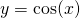we notice that the sign of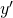around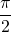is: +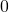- and thus there is a maximum. Similarly, the other point is a minimum.

0